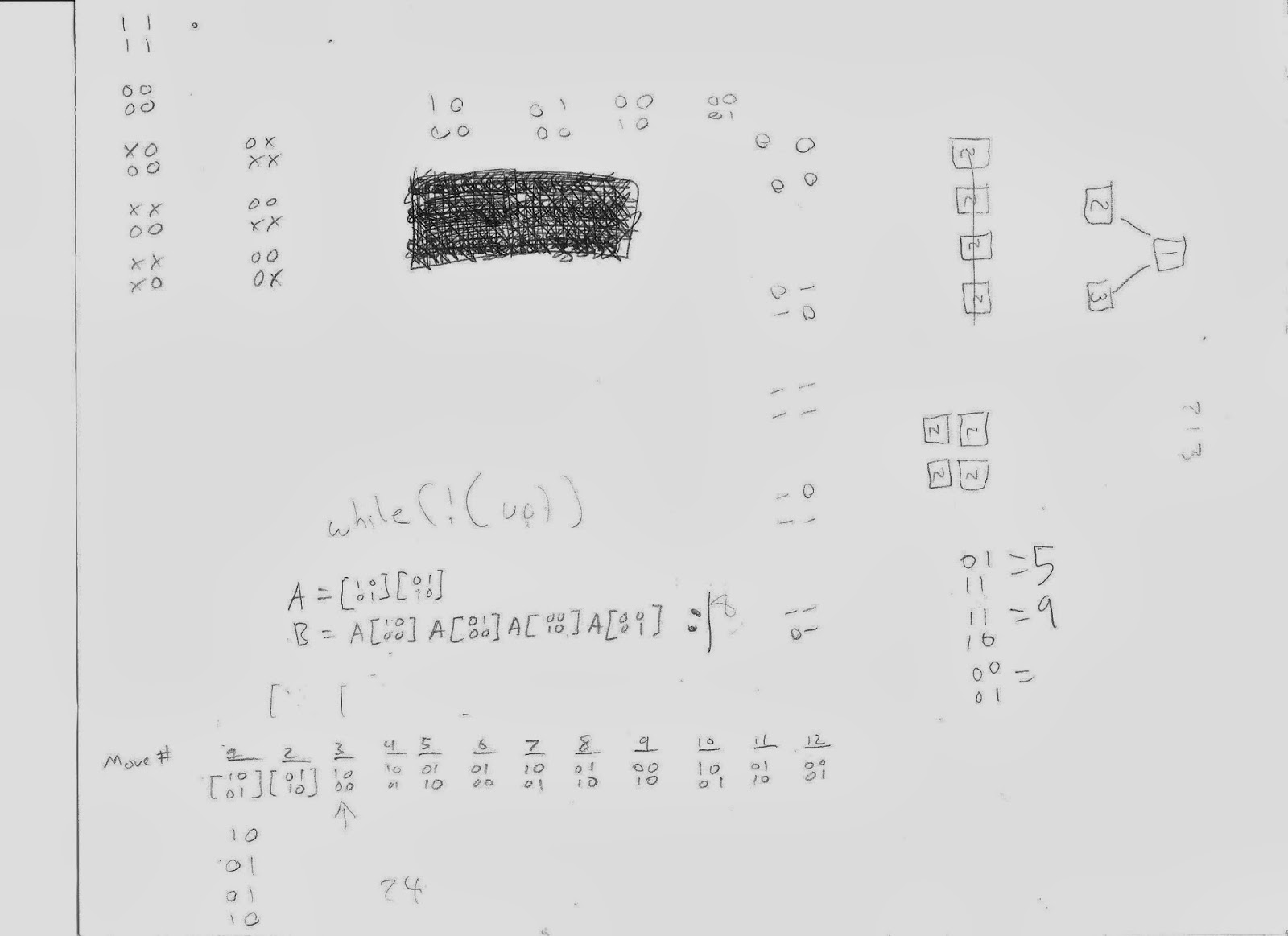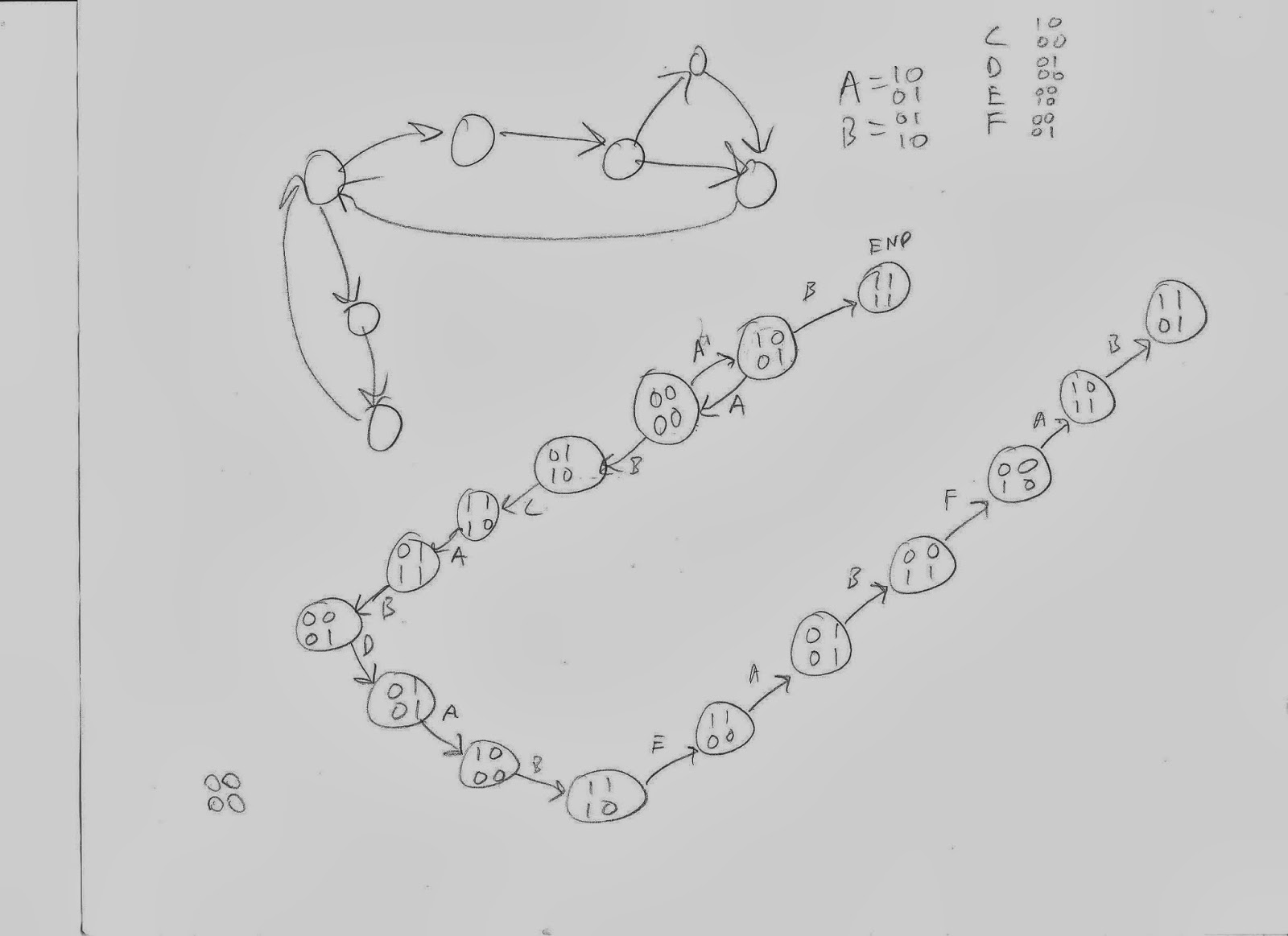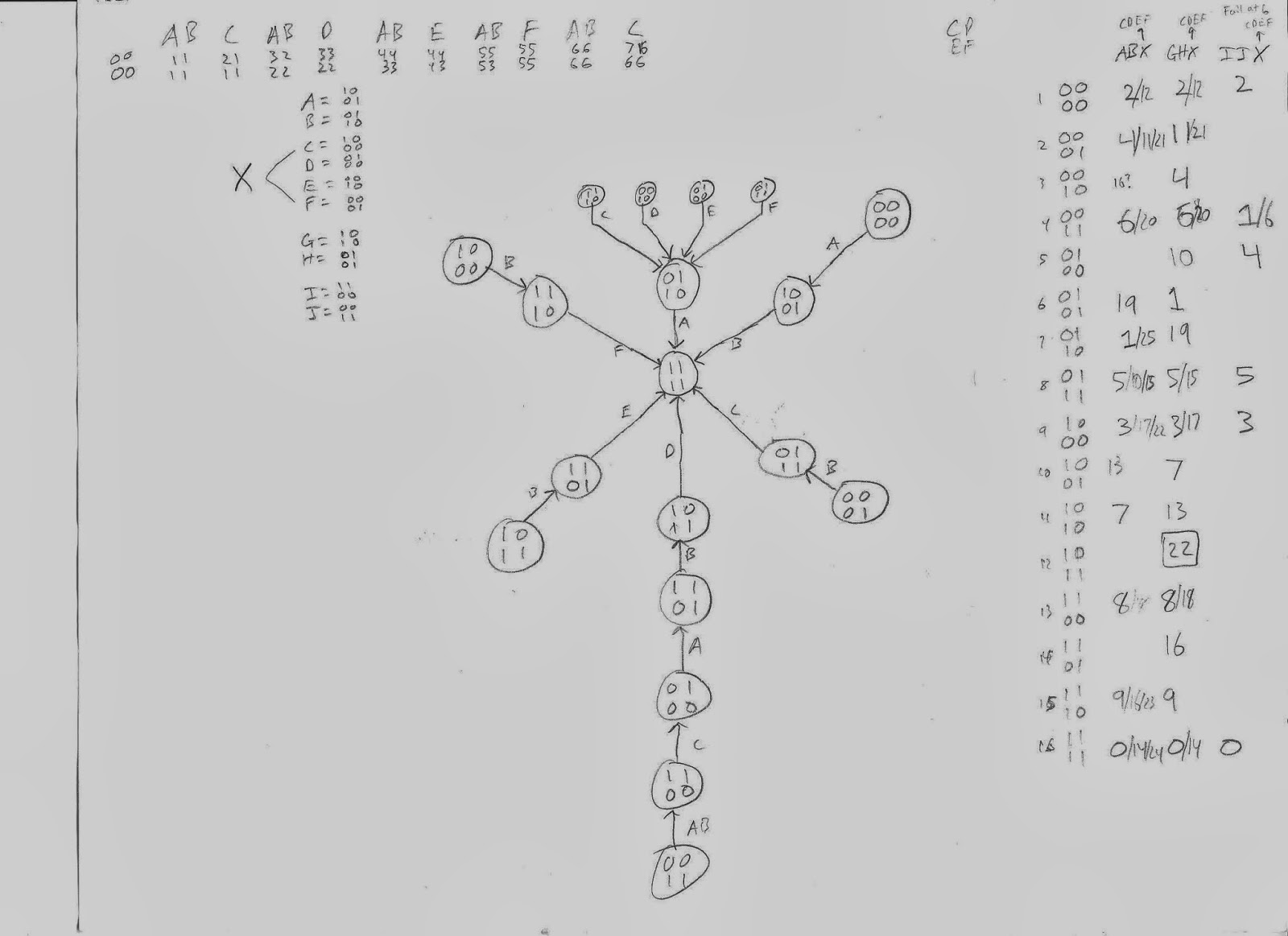## 2014-03-12

### Level Up - Puzzles: Four Random Cards, Turn All Face-Up, Blindfolded (Solution)

Problem:
You have four cards placed in a 2x2 grid pattern. Each card is randomly placed either face up or face down. Your goal is to flip all the cards face-up. But, there are a few restrictions:

• You are blindfolded and never know which cards are face up or face down. Nor do you know how many cards are flipped either way.
• Your movements for flipping cards are limited. Each turn you can flip either one or two cards.
• You will only be told when all cards are face up. You will get no other notification on how the cards are orientated.

What are the fewest amount of turns required to ensure all four cards are (eventually) flipped face up? And how?

Ascii art on how the layout may look. Each card represents a card:
1 2
3 4

Sidenote: When this brain teaser was first told to me last week, the person explained the possible limited card movements as, roughly, "you can either flip the diagonal cards, a column of cards, or a row of cards. Or, you can flip just a single card.". I'm pretty sure the way I explained it ("each turn, you can only flip one or two cards") is the same and much easier to understand, so I guess that lowers the puzzle difficulty a little bit for you, the reader.

Solution:

To put a larger buffer between the proposed question and answer, I will first write out some methods that didn't work. Then, at the very end of this blog post, I will give the final solution that I came up with.

When I first heard this problem, I was completely stumped, possibly because the problem wasn't explained that well. I just knew that there were 2^4 = 16 different possible combinations of cards that could appear on the table and had a theoretical lower limit of 15 moves to reach all 16 combinations. But, anyways, since I didn't know where or how to start, I just made a random guess on a simple pattern that might have worked. Or, if it didn't work, then it would have possibly let me learn more about the problem to have a better second guess.

At least, theoretically, that's how I would have approached the problem. In reality, my friend and I both heard the problem at the same time and he provided the first random idea/guess on how the problem may be solved.

Tangent:
In order to make the walkthroughs easier to explain, I will explain a few terms that I am going to use for the rest of this post.
Let the cards be arranged as
1 2
3 4
Let,
F1 mean flip just card 1,
F2 = flip card 2,
F3 = flip card 3,
F4 = flip card 4,
D1 = flip card 1 and 4 (D = diagonal)
D2 = flip card 2 and 3
C1 = flip card 1 and 3 (C = column)
C2 = flip card 2 and 4
R1 = flip card 1 and 2 (R = row)
R2 = flip card 3 and 4
END Tangent.

The first method tried was the pattern: D1, D2, F1, D1, D2, F2, D1, D2, F3, D1, D2, F4, then repeat until all cards were face-up. And, it seemed to work with the random first case of physical cards we used.

As my friend continued working on that method, I thought that if the D1, D2, F1-4 pattern (above) worked, then the C1, C2, F1-4 pattern and R1, R2, F1-4 pattern would work also, so I tried those methods. Since there were a relatively small number of possible outcomes, I created a chart that represented each of the 16 possible card layouts and manually calculated how many turns it would take to reach each of them from when all cards are face up.

One technique that I used for this brute forcing method was representing each possible type of turn as a 2x2 boolean matrix/array, with a "1" meaning flip the card and "0" meaning stay (aka don't flip) . Then, it was easier to see everything mathematically, and in the problem's truest (simplest/logical) form. Also, I had an intuition that the order of the flips didn't matter, and by representing the moves as matrices, I know that there is some theorem or axiom that says adding matrices is commutative.

So, using brute force methods I was able to determine that the C1, C2, F1-4 pattern worked eventually. But, it is possible that I made a mistake, so that solution wasn't good enough for me because I wanted to figure out a real proof and have a better line of reasoning on why the pattern worked or not.

My first idea for creating a real proof to the problem was drawing a FSM (Finite State Machine) . Then, after that started getting messy, I drew a much nicer and organized FSM  which helped. As I was drawing the second FSM, I learned more on the way the different patterns worked and came up with a diagram that actually worked . But, to make a long story not as long, I suddenly had a breakthrough on another method was much simpler to draw and explain.

My breakthrough on the problem was that each of the 16 different possible card combinations could be drawn on a Karnaugh map , which I probably first learned in my Logic Design class a few years ago. The beauty of a Karnaugh map is that it is basically based on Gray code. Though, instead of putting output in the boxes, I just put the values that represented what the cards would look like at each instance. That way, I had a visual representation of what moves I could make and see some patterns more easily.

Now that the array of possible card layouts is drawn , it is organized in such a way that if you move up/down/left/right on the grid, then it only requires changing one card at a time. Thus, a solution is found for the theoretical minimum of this problem. The following pattern visits each of the different possible layouts one time each, with no repeats, and you can start from any random card arrangement:
F1,F2,F1,F3,  F1,F2,F1,F4,  F1,F2,F1,F3,   F1,F2,F1 [,F4]
The above pattern just visits all each node in a row, then changes rows, then repeats. Using another pattern of single flips you can zig-zag differently across the entire board  in a non-symmetrical pattern from the one given explicitly above.

As I examine the 2-D arrangement of possible combinations, I can see that there are other patterns, as well, which use the 2-card movements (C1/2, D1/2, and/or R1/2). I leave the task of finding those full patterns to the reader. Hint: In the K-map, if you do it right, you can move diagonally and more than one space horizontally/vertically.First time figuring out problem, representing problem in matrices.The top-left has a sample FSM (Finite State Machine), the other larger "FSM" was my first try at trying to represent the problem in a state diagram.My second try at a state diagram. On the right are the number of moves it takes to reach each face up from that layout (but, there are a few errors there).My third try at an organized state diagram. It works, but it's not pretty. The square represents the card layout that will be solved in X number of moves, where X is the number of the far left and right side. The fallback using this method is that it would take a lot of work to calculate each of the values at the square and can't be too sure that there are no repeats at the squares.The only diagram needed to solve this 4-card brain teaser. Using this particular organization with the influence of Gray code is all that is needed to find the multiple patterns that require the theoretical minimum of 15 moves to see all 16 results.

This has just been my logical/computational studies that lead me to the solution explained this way. If there is a read that can explain this puzzle another way, then I'd be happy to hear it.

Happy Puzzling!

~ Danial Goodwin ~